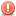CodeGuru Home VC++ / MFC / C++ .NET / C# Visual Basic VB Forums Developer.com

# Thread: help need proper equation!

1.Junior MemberJoin Date
Jan 2009
Posts
6

##help need proper equation!

For this program I have to obtain the variance. The variance is obtained by subtracting the calcAverage from each value in testvals, squaring the values obtained, adding them, and dividing by the number of elements in testvals. Basically the last line of code I need help because I can't figure out how to do all the math with one formula. This is the formula I need help with:

Code:
`variance = ((testvals[i] - (testvals, MAXELS)));`
I know thats not right obviously. So any help would be greatly appreciatedHeres what I have so far:

Code:
```#include <iostream>
#include <iomanip>
#include <cmath>

using namespace std;

const int MAXELS=14;
float calcAverage(int [MAXELS]); //function prototype
float variance(int [MAXELS]); //function prototype
int main()
{
int testvals[MAXELS] = {89, 95, 72, 83, 99, 54, 86, 75, 92, 73, 79, 75, 82, 73};
cout << "The average of these numbers is: " <<calcAverage(testvals) <<endl;
cout << "The variance of these number is: " <<variance(testvals) <<endl;
system("pause");
return 0;
}
//find the average value
float calcAverage(int testvals[MAXELS])
{
int i;
float calcAverage, total=0;
for(i=0; i < MAXELS; i++)
{
total=total+testvals[i];
}
calcAverage=total/MAXELS;
return calcAverage;
}

//find the variance
float variance(int testvals[MAXELS])
{
int i;
float calcAverage, sqd, variance=0;
for (i=0; i < MAXELS; i++)
{
variance = ((testvals[i] - (testvals, MAXELS)));
}
return variance;
}```

Last edited by Marc G; February 3rd, 2009 at 04:07 AM. Reason: Added code tagsReply With Quote

2.MemberJoin Date
Jun 2005
Posts
315

## Re: help need proper equation!

I can't figure out how to do all the math with one formula
Is doing the calculation in multiple steps not an option?Reply With Quote

3.Junior MemberJoin Date
Jan 2009
Posts
6

## Re: help need proper equation!

Actually it is an option but I just don't know how to do it at all.Reply With Quote

average, c++, equation, formula, variance####Posting Permissions

• You may not post new threads
• You may not post replies
• You may not post attachments
• You may not edit your posts
•